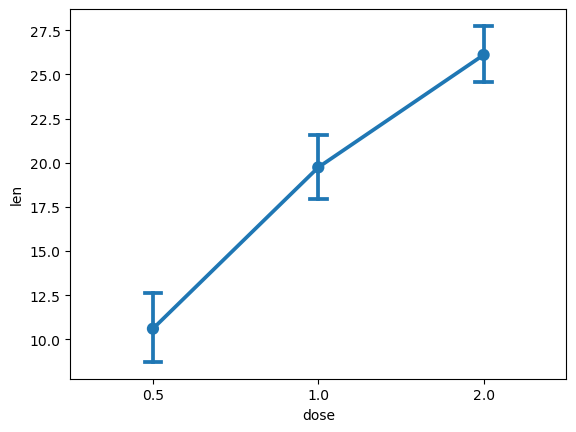# How to analyze the sample means of different treatment conditions (in Python, using Matplotlib and Seaborn)

See all solutions.

In a single-factor experiment with three or more treatment levels, how can we compare them to see which one impacts the outcome variable the most?

## Solution

The solution below uses an example dataset about the teeth of 10 guinea pigs at three Vitamin C dosage levels (in mg) with two delivery methods (orange juice vs. ascorbic acid). (See how to quickly load some sample data.)

1
2
from rdatasets import data
df = data('ToothGrowth')


To visually plot the means of the length of the tooth based on the Vitamin C dosage levels we can create a pointplot. We will have to import the seaborn and matplotlib.pyplot packages to be able to create it.

1
2
3
4
5
6
import seaborn as sns
import matplotlib.pyplot as plt
sns.pointplot( x = 'dose', y = 'len', data = df,
ci = 95,         # ci stands for Confidence Interval
capsize = 0.1 )  # the width of the "caps" on error bars
plt.show()

1
2
3
4
5
/tmp/ipykernel_4429/664134235.py:3: FutureWarning:

The ci parameter is deprecated. Use errorbar=('ci', 95) for the same effect.

sns.pointplot( x = 'dose', y = 'len', data = df,The point plot informs us that as the dosage levels increase, the tooth length also increases.

To obtain the actual numbers, we can use the groupby function to compute the treatment level means, and the mean function to compute the mean for the entire column.

1
df.groupby('dose')['len'].mean()

1
2
3
4
5
dose
0.5    10.605
1.0    19.735
2.0    26.100
Name: len, dtype: float64

1
df['len'].mean()

1
18.813333333333336


If you wish to display the difference between the overall mean and the group means, you can subtract the overall mean from the treatment level means.

1
df.groupby('dose')['len'].mean() - df['len'].mean()

1
2
3
4
5
dose
0.5   -8.208333
1.0    0.921667
2.0    7.286667
Name: len, dtype: float64